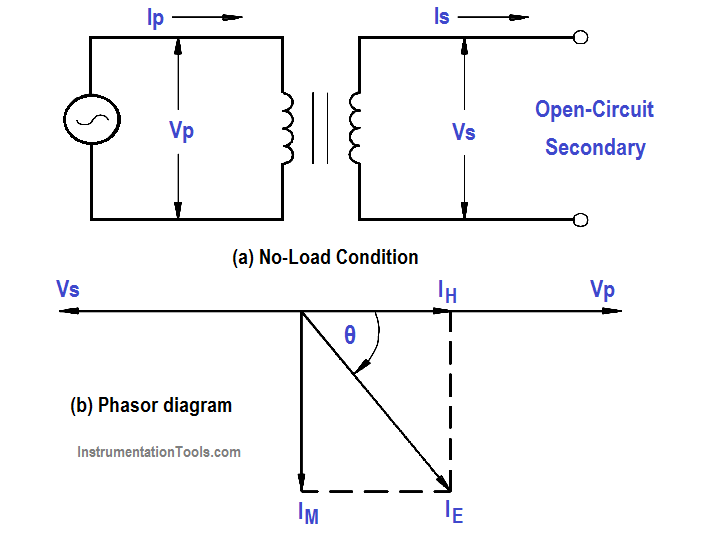If the secondary of a transformer is left open-circuited (Figure 6), primary current is very low and is called the no-load current. No-load current produces the magnetic flux and supplies the hysteresis and eddy current losses in the core.

The no-load current (IE) consists of two components: the magnetizing current (Im ) and the core loss (IH). Magnetizing current lags applied voltage by 90°, while core loss is in phase with the applied voltage (Figure 1b). VP and VS are shown 180° out of phase. IH is very small in comparison with Im , and Im is nearly equal to IE . No-load current, IE , is also referred to as exciting current.Figure 1 : Open-Circuit Secondary

Example:

When the secondary of a 120/440 V transformer is open, primary current is 0.2 amps at a PF of .3. The transformer is a 5 kVA transformer. Find: (a) IP ,  (b) IE   (c) IH , and (d) Im

Solution :

(a) Full Load Current = KVA Rating / Vp

(b) IP at no load current is equal to IE

(c) IH = IE Cos θ = IE  x PF

IH = 0.2 x 0.3 = 0.06 amps

(d) Im = IE Sin θ

θ = across 0.3 = 72.5°

I= 0.19 amps